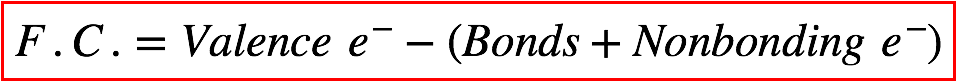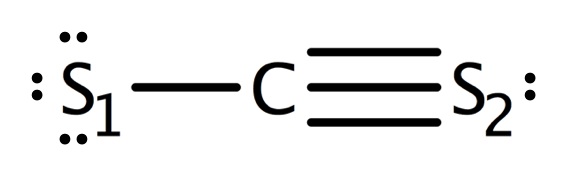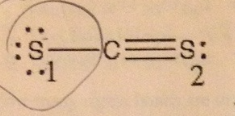# Problem: Given the Lewis Structure shown below, what is the formal charge on the sulfur atom labeled #1?A. 0B. -1C. +1D. -2E. +2

###### FREE Expert Solution
100% (353 ratings)
###### FREE Expert Solution

We’re being asked to determine the formal charge of sulfur (labeled #1) in the given Lewis structure. Recall that the formula for the formal charge is:The given Lewis structure is:100% (353 ratings)###### Problem Details

Given the Lewis Structure shown below, what is the formal charge on the sulfur atom labeled #1?

A. 0
B. -1
C. +1
D. -2
E. +2What scientific concept do you need to know in order to solve this problem?

Our tutors have indicated that to solve this problem you will need to apply the Formal Charge concept. You can view video lessons to learn Formal Charge. Or if you need more Formal Charge practice, you can also practice Formal Charge practice problems.

What is the difficulty of this problem?

Our tutors rated the difficulty ofGiven the Lewis Structure shown below, what is the formal ch...as low difficulty.

How long does this problem take to solve?

Our expert Chemistry tutor, Sabrina took 2 minutes and 20 seconds to solve this problem. You can follow their steps in the video explanation above.

What professor is this problem relevant for?

Based on our data, we think this problem is relevant for Professor Reed's class at University of Guelph.